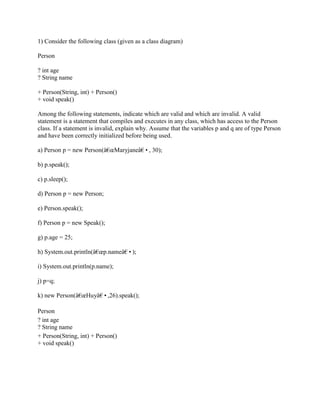Diese Präsentation wurde erfolgreich gemeldet.

# 1) Consider the following class (given as a class diagram) Person - in.docx

Anzeige
Anzeige
Anzeige
Anzeige
Anzeige
Anzeige
Anzeige
Anzeige
Anzeige
Anzeige
Anzeige×

1 von 3 Anzeige

# 1) Consider the following class (given as a class diagram) Person - in.docx

1) Consider the following class (given as a class diagram)
Person
? int age
? String name
+ Person(String, int) + Person()
+ void speak()
Among the following statements, indicate which are valid and which are invalid. A valid statement is a statement that compiles and executes in any class, which has access to the Person class. If a statement is invalid, explain why. Assume that the variables p and q are of type Person and have been correctly initialized before being used.
a) Person p = new Person(â€œMaryjaneâ€, 30);
b) p.speak();
c) p.sleep();
d) Person p = new Person;
e) Person.speak();
f) Person p = new Speak();
g) p.age = 25;
h) System.out.println(â€œp.nameâ€);
i) System.out.println(p.name);
j) p=q;
k) new Person(â€œHuyâ€,26).speak();
Solution
public class Person {

int age;
String name;
public static void main(String[] args)
{

Person p = new Person(\"Maryjane\", 30);
Person q = new Person(\"Maryjane\", 30);
p.speak();
p.sleep();
//Person p = new Person;
// Person.speak();
//Person p = new Speak();
p.age = 25;
System.out.println(\"p.name\");
System.out.println(p.name);
p=q;
new Person(\"Huy\",26).speak();
}
Person(){}
public Person(String string, int i) {
// TODO Auto-generated constructor stub
}
void sleep(){

}
void speak(){

}
}
invalid statement
Person p = new Person;
Person.speak();
Person p = new Speak();
.

1) Consider the following class (given as a class diagram)
Person
? int age
? String name
+ Person(String, int) + Person()
+ void speak()
Among the following statements, indicate which are valid and which are invalid. A valid statement is a statement that compiles and executes in any class, which has access to the Person class. If a statement is invalid, explain why. Assume that the variables p and q are of type Person and have been correctly initialized before being used.
a) Person p = new Person(â€œMaryjaneâ€, 30);
b) p.speak();
c) p.sleep();
d) Person p = new Person;
e) Person.speak();
f) Person p = new Speak();
g) p.age = 25;
h) System.out.println(â€œp.nameâ€);
i) System.out.println(p.name);
j) p=q;
k) new Person(â€œHuyâ€,26).speak();
Solution
public class Person {

int age;
String name;
public static void main(String[] args)
{

Person p = new Person(\"Maryjane\", 30);
Person q = new Person(\"Maryjane\", 30);
p.speak();
p.sleep();
//Person p = new Person;
// Person.speak();
//Person p = new Speak();
p.age = 25;
System.out.println(\"p.name\");
System.out.println(p.name);
p=q;
new Person(\"Huy\",26).speak();
}
Person(){}
public Person(String string, int i) {
// TODO Auto-generated constructor stub
}
void sleep(){

}
void speak(){

}
}
invalid statement
Person p = new Person;
Person.speak();
Person p = new Speak();
.

Anzeige
Anzeige

### 1) Consider the following class (given as a class diagram) Person - in.docx

1. 1. 1) Consider the following class (given as a class diagram) Person ? int age ? String name + Person(String, int) + Person() + void speak() Among the following statements, indicate which are valid and which are invalid. A valid statement is a statement that compiles and executes in any class, which has access to the Person class. If a statement is invalid, explain why. Assume that the variables p and q are of type Person and have been correctly initialized before being used. a) Person p = new Person(â€œMaryjaneâ€•, 30); b) p.speak(); c) p.sleep(); d) Person p = new Person; e) Person.speak(); f) Person p = new Speak(); g) p.age = 25; h) System.out.println(â€œp.nameâ€•); i) System.out.println(p.name); j) p=q; k) new Person(â€œHuyâ€•,26).speak(); Person ? int age ? String name + Person(String, int) + Person() + void speak()
2. 2. Solution public class Person { int age; String name; public static void main(String[] args) { Person p = new Person("Maryjane", 30); Person q = new Person("Maryjane", 30); p.speak(); p.sleep(); //Person p = new Person; // Person.speak(); //Person p = new Speak(); p.age = 25; System.out.println("p.name"); System.out.println(p.name); p=q; new Person("Huy",26).speak(); } Person(){}
3. 3. public Person(String string, int i) { // TODO Auto-generated constructor stub } void sleep(){ } void speak(){ } } invalid statement Person p = new Person; Person.speak(); Person p = new Speak();